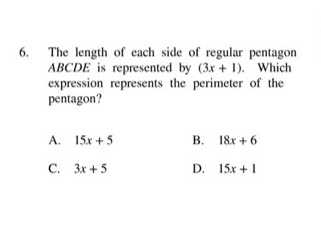### ¿Todavía tienes preguntas de matemáticas?

Pregunte a nuestros tutores expertos
Algebra
Pregunta6. The length of each side of regular pentagon

$$A B C D E$$ is represented by $$( 3 x + 1 )$$ . Which expression represents the perimeter of the pentagon?

A. $$15 x + 5$$ B. $$18 x + 6$$

C. $$3 x + 5$$ D. $$15 x + 1$$